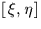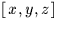Next: SLA_DTP2V - Tangent Plane to Direction Cosines
Up: SUBPROGRAM SPECIFICATIONS
Previous: SLA_DTF2R - Hour,Min,Sec to Radians

## SLA_DTP2S - Tangent Plane to Spherical

ACTION:
Transform tangent plane coordinates into spherical coordinates (double precision)

CALL:
CALL sla_DTP2S (XI, ETA, RAZ, DECZ, RA, DEC)

GIVEN:

 D XI,ETA tangent plane rectangular coordinates (radians) RAZ,DECZ D spherical coordinates of tangent point (radians)

RETURNED:

NOTES:
1.
The projection is called the gnomonic projection; the Cartesian coordinatesare called standard coordinates. The latter are in units of the distance from the tangent plane to the projection point, i.e. radians near the origin.
2.
When working inrather than spherical coordinates, the equivalent Cartesian routine sla_DTP2V is available.Next: SLA_DTP2V - Tangent Plane to Direction Cosines
Up: SUBPROGRAM SPECIFICATIONS
Previous: SLA_DTF2R - Hour,Min,Sec to Radians

SLALIB --- Positional Astronomy Library